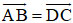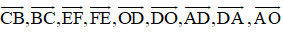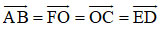# Solving geometry 10 pages 7 lessons 1, 2, 3, 4

253 total views

2021-07-21 16:51:43

The following article will help you solve exercises 1, 2, 3, 4 on page 7 of the 10th grade geometry textbook. These are exercises to help you master the concept of vectors, vectors of covariance, vectors. same direction, two vectors are equal, vector 0. These are the basic knowledge to help you solve other basic and advanced geometry exercises, please refer.

## Solving geometry 10 pages 7 lessons 1

Given three vectors a →, b →, c → all different vectors . The following statements true or false?

a) If two vectors a→, b→ have the same direction as c→, then a→ and b→ have the same direction.

b) If a→, b→ are in the same direction as c→, then a→ and b→ are in the same direction.

a) Let Δ1, Δ2, Δ3 be the prices of the three vectors a→, b→, c→ ., respectively

+ Vector a has the same direction as vector c Δ1 //≡ Δ3

+ Vector b has the same direction as vector c Δ2 //≡ Δ3

1 //≡ 2

⇒ The vector a→ is in the same direction as b→ (by definition).

b) a→, b→ in the same direction as c→

⇒ a→, b→ are in the same direction as c→

⇒ a → and b → are in the same direction.

⇒ a→ and b→ can only be in the same direction or in the opposite direction.

Since a → and b → are both in the opposite direction of c → so a → and b → have the same direction.

## Solution 2 page 7 Geometry 10

In Figure 1.4, show the vectors in the same direction, the same direction, the opposite direction, and the vectors are equal.

– Vectors in the same direction:

a→ and b→ the same direction

u→ and v→ are in the same direction

x→, y→, w→ and z→ are in the same direction.

– Vectors in the same direction:

a→ and b→ in the same direction

x→, y→ and z→ in the same direction

– Reverse vectors:

u→ and v→ opposite direction

w→ opposite direction to the vectors x→, y→ and z→

– Equal vectors: x → = y

## Solving geometry 10 lessons 3 pages 7

Given quadrilateral ABCD. Prove that the quadrilateral is a parallelogram if and only if## Solving geometry 10 lessons 4 pages 7

Let ABCDEF be a regular hexagon with center O.

a) Find vectors other than O → and in the same direction as OA →.

b) Find vectors using vector AB →a) Vectors other than O → and in the same direction as OA → are:b) Vectors equal to vector AB → are:Above is the solution to Lesson 1, 2, 3, 4, page 7 of Grade 10 Geometry Textbook. If you have any questions or suggestions, please leave a comment at the end of the article.

.

#Solving #geometry #pages #lessons

Share